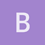Archive 04/01/2022.

Invariants vs ML (as per Casanellas-Rius and Felsenstein)ematsen

The most recent phyloseminar is a very nice intro to phylogenetic invariants by Marta Casanellas-Rius, along with updates about her Erik+2 method.

It started a discussion over email between her and Joe Felsenstein, and I thought I’d copy the discussion here.

This is Marta’s response, so Joe’s original questions are shaded.

Here’s the figure she describes as a PDF:

I think that Dr. Casanellas-Rius has made a good case for the usefulness of edge-invariants distance methods in cases where there are mixtures of sets of edge lengths or other models that are not easy to use in ML (or Bayesian) approaches.

I just want to defensively quibble about one thing. I think that in the case where we have a single model (not a mixture of different edge lengths) we have statistical theorems, dating back to RA Fisher, guaranteeing the asymptotic efficiency of ML methods. So in theory ML should do best in asymptopia.

Yes, let’s stick to unmixed (also in the attached file I don’t consider mixtures). Although ML could do best in theory (and asymptotically speaking), there is always the issue that in practice we only get local maxima and not global maxima and this is why ML is not a perfect method. This problem of local maxima gets worse when the are more parameters to estimate and for example, performing ML to a GMM tree can result in incorrect results. So I admit that yes, ML should be the best but in practice is not, speccially for very general models as GMM.

Use of the full set of invariants should be equivalent. Why did it do worse in Dr. Casanellas-Rius’s simulations? I suggest that it is because the measure used to judge degree of fit was not equivalent to the one ML uses. That latter is something like a Kullback-Leibler distance on the pattern probabilities. If one would use that one, there would then actually be no difference between ML and full-invariants.

I agree that using a “full” set of invariants is statistically consistent that is, when the length of the alignment tends to infinite, this set of invariants allow to recover the true tree. Actually, inseatd of using the “full” set of invariants I’d use a “local complete intersection” (see the attached explanation). So, yes, asymptotically and if ML implementation got global maxima, there should be no difference between ML and a “local complete intersection”.

However, I sheepishly acknowledge that this measure for full-invariants methods has not, as far as I know, been developed.

Yes, this was developed in Casanellas, Fernandez-Sánchez, MBE 2007

but you can have a look at the figure I attach in order to see the sifference in performance between full set of invariants and edge invariants.

(And there would be no reason to spend a lot of time developing it, if one could achieve the same inference by just using ML).

As I said, in practice one cannot achieve the same inference of ML due to the issue of local maxima and complex models (although I must also say that invariants also have drawbacks).felsenst

In principle ML will be equivalent to using a full set of invariants, if one has the proper measure of goodness of fit. However such a measure has not been developed. The measure developed in the Casanellas et al paper that was cited here is not the measure that is equivalent to use of ML. It is a sum of absolute values of the invariants. The use of absolute values (instead of squares, or fourth powers, or absolute values of sines, or whatever) is a reasonable arbitrary choice. But in principle there is a measure that is equivalent to ML (in principle, but not developed in practice yet). If that existed, it would be statistically efficient. The Casanellas et al method is not.

I am actually not arguing about consistency – whether the estimation would converge to the truth with large amounts of data. I am arguing about efficiency. Whether the variances and covariances of the branch lengths are at a minimum. Technically, ML achieves that minimum, though only asymptotically. An invariants method that uses some other method of goodness of fit is not equivalent to ML, and therefore does not achieve efficiency.

I’m not saying that the present invariants method is a bad one, I’m just talking about whether it is 100% efficient.felsenst

I think that an optimal measure of the distance of the set of invariants from zero could (in principle) be developed by having expressions for the covariances of the values of the invariants, and using those to make a Mahalanobis distance. I’m not claiming that this can be done in practice!Marta_Casanellas

Indeed, an optimal measure has not yet been developed (in our paper in MBE we used a naive approach only). I agree.

We have to take into account that the number of invariants in a “full-set” is exponential in the number of leaves (and there are infinitely many “full-sets” of invariants, one should make a choice). Also, the number of invariants in a “local complete intersection” is exponential. Even more: the number of edge invariants is still exponential. This is the reason why the most recent methods based on invariants do not try to get an optimal measure but, instead, have focused on evaluating the distance from matrices to the set of rank 4 matrices. However, an optimal measure for this other score has not yet been developed either.